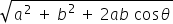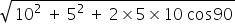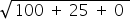science
Easy

Question

# If a car moves 10 m in the north direction and 5 m in the west direction, then find the resultant.

## 11.1821.316.512.3Hint:

## The correct answer is: 11.18

### If two vectors A and B with magnitude a and b respectively are given and the angle between them is θ Then the magnitude of the Resultant of A and B is R =So R =R =R = 11.3 (approx)#### With Turito Foundation.#### Get an Expert Advice From Turito.